# Factorial of a Number in C

## Factorial of a Number in C Language with Examples:

In this article, I am going to discuss factorial of a number in C Language with Examples by using the following ways:

1. Loop
2. Recursion

Let’s discuss each way with a detailed explanation.

##### Factorial of a Number in C Language By using Loop:

In the below example, we will calculate the factorial of a number by using for loop. In the main function, first, we declare two variables v and fact (with a value of 1). We here initialize fact variable with value one not with zero as the result of whole multiplication will be zero. On the next line, we give some value to the variable v which will find the factorial of that value. Then, we run our for loop and inside this loop, we add a statement “fact = fact * i” which modifies our fact variable. And the last statement is the print statement which prints our factorial on the screen.

```#include <stdio.h>
int main() {
int v, fact;
fact = 1;
v = 4;

for (int i = 1; i <= v; i++) {
fact = fact * i;
}
printf("%d", fact);
}
```

Output: 24

Time Complexity: O(n)

##### Factorial of a Number in C Language By using Recursion:

We will find the factorial of a number with recursion. Let’s understand each and every step in a detailed explanation. In the below example, we created a function fact that takes one parameter. In the next step, we define our base condition (where the function terminates or ends) which is if v == 0. If it satisfies then return 1 otherwise return fact (v – 1) * v.

In this fact function, the value will be returned at returning time from recursive calls. This function will terminate at v == 0. And when it terminates it returns 1, from there it starts multiplying this 1 with function parameters i.e., 1 * 2 * 3 * 4. And in this manner, our recursive function fact will calculate the factorial of the given value.

```#include <stdio.h>
int fact(int v) {
if (v == 0)
return 1;
return fact(v - 1) * v;
}

int main() {
int v = 4;
printf("%d", fact(v));
}
```

Output: 24

Time Complexity: O(n)

##### Tracing Tree of the above Factorial Number using Recursion:

Now, Let’s jump on the Tracing Tree where we will understand each and every step.

##### Step1: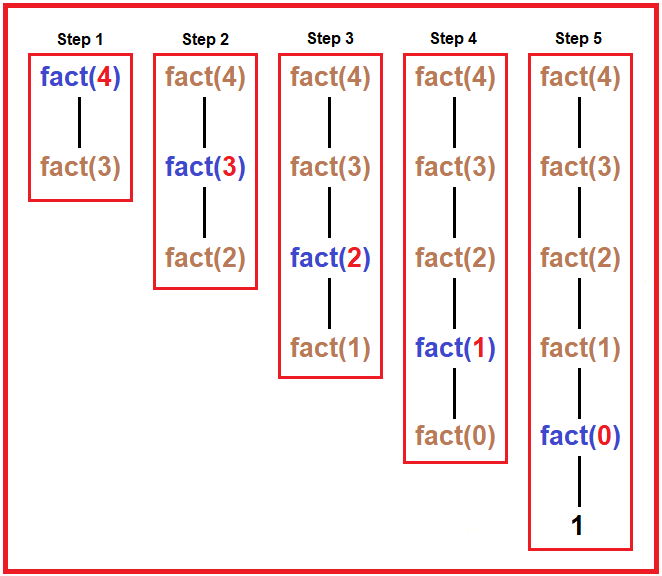Tree – In the above diagram, there are 5 steps. In every step, we have shown every recursive call of fact function. As shown in the diagram, we passed 4 as a parameter.

1. In 1st step, it checks if 4 == 0? No, then it goes to the else part which returns fact (4 – 1) * 4. Here we can’t perform multiplication, first, it has to finish fact (4 – 1) call then only multiplication will perform.
2. In 2nd step, it checks if 3 == 0? No, then it goes to the else part which returns fact (3 – 1) * 3. Here also we will have to first calculate the result of fact (3 – 1) call then only multiplication will perform.
3. In 3rd step, it checks if 2 == 0? No, then it goes to the else part which returns fact (2 – 1) * 2. we will have to first calculate the result of fact (2 – 1) call then only multiplication will perform.
4. In 4th step, it checks if 1 == 0? No, then it goes to the else part which returns fact (1 – 1) * 1. we will have to first calculate the result of fact (1 – 1) call then only multiplication will perform.
5. In 5th step, it checks if 0 == 0? Yes, then it returns 1. And from here multiplication starts, returning time from recursive calls. Now it will multiply the result of each recursive call with the function parameter and at the final call, it will finally return the calculated value of factorial of the given value.
##### Step2: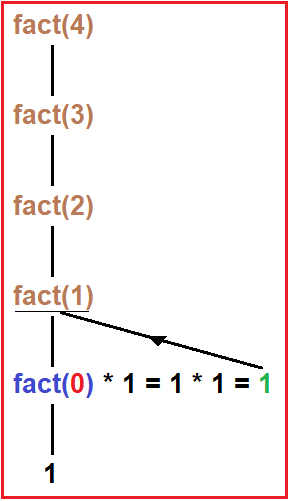Tree – Now as we discussed in the previous step when the base condition (v == 0) true then it will return 1. And this 1 will return to the previous call and be multiplied by the function parameter as shown in the diagram, here the result of the multiplication is 1 which further returns to the previous recursive call.

##### Step3: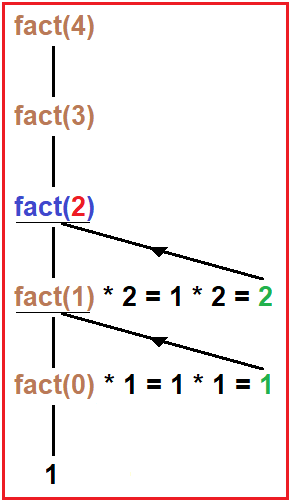Tree – Now the multiplied value is 2 which returns to the fact (3) call and is further multiplied by function parameter i.e., 3.

##### Step4: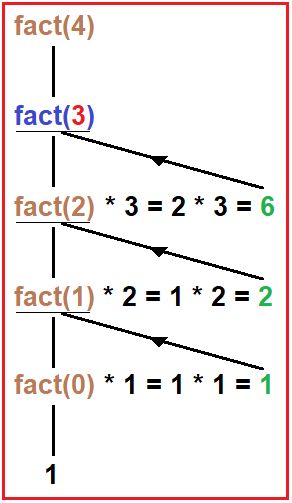Tree – Now the calculated value is 6 which returns to the fact (4) call and here again multiplication takes place between 6 and 4.

##### Step5: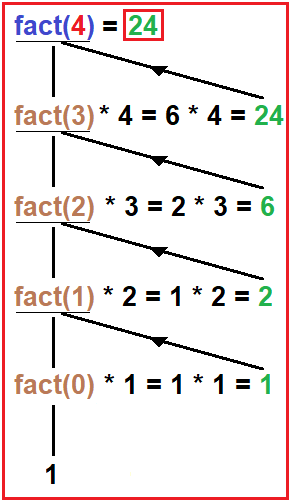Tree – Now the final calculated value Is 24 which fact (4) returns to that place where we have called this inside the main function. In our current example, we call this function in the print statement. Now, as the function return the final value it will print on the output screen.

In the next article, I am going to discuss Power using Recursion in C Language with Examples. Here, in this article, I try to explain the Factorial of a number in C Language with Example and I hope you enjoy this Factorial of a number in C Language with Example article.